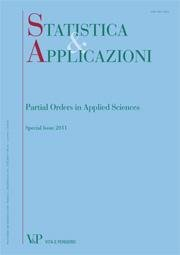Your browser does not support JavaScript!

Hans-Georg Bartel

Author's books

Finding incomparable pairs of subsets by using Formal Concept Analysis
Free
digitalformat:Article| STATISTICA & APPLICAZIONI - 2011 - Special issue. Partial orders in applied sciences
Year: 2011
In the paper presented here, we use Formal Concept Analysis (FCA) to solve a problem that arises when working with partially ordered sets (posets). In detail, the task here is to look for incomparable subsets which are related to a given poset. A way to solve this problem is to use FCA based on a context which can be derived in some steps from the _-matrix of the (simple directed) graph corresponding to the given poset. The requested incomparable subsets result from the set of concepts obtained from this context. For illustrative purposes, small toy data sets are presented. At the end, a real data application to environmental chemistry is given in detail. The data consist of ten chemicals found in the German river Main. As the result a set of twelve incomparable pairs of subsets are figured out. Keywords: Bipartite Graph, Adjacency Matrix, Formal Concept Analysis, Partially Ordered Set, Incomparability of Sets.